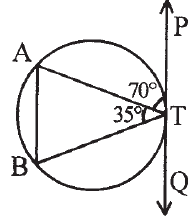# SilverZone - IOM PDF Sample Papers for Class 10

Class 10 sample paper & practice questions for International Olympiad of Mathematics (IOM) level 1 are given below. Syllabus for level 1 is also mentioned for these exams. You can refer these sample paper & quiz for preparing for the exam.#### Resources:

##### Sample Questions from Olympiad Success:
 Q.1 Q.2 Q.3 Q.4 Q.5 Q.6 Q.7 Q.8 Q.9 Q.10
 Q.1 Ankit and Anuj started simultaneously from X and Y respectively. Each person started towards the starting point of the other XY = 18 km. The speeds of Ankit and Anuj throughout their journeys were 5 kmph and 4 kmph respectively. On reaching X or Y, each turned back immediately to resume the journey to his starting point. Find the total distance travelled by Ankit before meeting Anuj for the second time. (in m) a) 30 b) 27 c) 24 d) 33
 Q.2 A jar containing a mixture of milk and water has 55% milk. A part of this mixture is replaced by another mixture of milk and water having 20% milk. If now the mixture has 35% milk, what fraction of the jar was not replaced? a) 3/4 b) 4/7 c) 3/7 d) 4/3
 Q.3 What is the value of D in the equation 9x² + 15x + 4 = 0? a) 125 b) 49 c) 81 d) 100
 Q.4 Look at the image given below and find the value of ∠BTQ.a) 30⁰ b) 45⁰ c) 75⁰ d) 90⁰
 Q.5 If f(x) = x² – 5x – 14, find the value of f(7). a) -1 b) 1 c) 0 d) 3
 Q.6 At Bilal’s Discount Hardware everything is sold for 30% less than the market price. If Bilal’s buys tool bits for Rs. 96, what price should he marked them if he wants to make a 20% profit on his cost? a) 117 b) 127 c) 146 d) 164
 Q.7 6x – 3y + 10 = 0, 2x – y + 9 = 0 represents two lines which are: a) Intersecting at exactly one point b) Intersecting at exactly two points c) Coincident d) Parallel
 Q.8 A tangent to a circle (centre O) drawn from an exterior point T to the circle and touches the circle at a point P on the circumference. Which of the following statement is TRUE? a) ∠TPO > 90° b) ∠TPO < 180° c) ∠TPO < 90° d) ∠TPO = 90°
 Q.9 Every number on the number line represents a ________ number which is either _________ or _________. a) Real, Rational, Irrational b) Complex, Rational, Irrational c) System, Rational, Irrational d) None of these
 Q.10 Which of these is false? a) The diagonal of the parallelogram divide the parallelogram into four equal parts b) Area of parallelogram is base multiplied by the height c) If EFGH are the respective midpoints of the sides of the parallelogram ABCD, Area of EFGH is half the area of the parallelogram d) None of theseSample PDF of SilverZone - International Olympiad of Mathematics (IOM) PDF Sample Papers for Class 10:

 Q.1 )a Q.2 )a Q.3 )c Q.4 )c Q.5 )c Q.6 )d Q.7 )d Q.8 )d Q.9 )a Q.10 )d

Q.1 : a | Q.2 : a | Q.3 : c | Q.4 : c | Q.5 : c | Q.6 : d | Q.7 : d | Q.8 : d | Q.9 : a | Q.10 : d